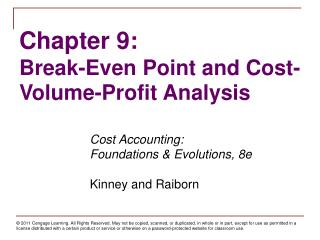# Chapter 9: Break-Even Point and Cost-Volume-Profit Analysis - PowerPoint PPT PresentationDownload PresentationChapter 9: Break-Even Point and Cost-Volume-Profit Analysis

Chapter 9: Break-Even Point and Cost-Volume-Profit AnalysisDownload Presentation## Chapter 9: Break-Even Point and Cost-Volume-Profit Analysis

- - - - - - - - - - - - - - - - - - - - - - - - - - - E N D - - - - - - - - - - - - - - - - - - - - - - - - - - -
##### Presentation Transcript

1. Chapter 9:Break-Even Point and Cost-Volume-Profit Analysis Cost Accounting: Foundations & Evolutions, 8e Kinney and Raiborn

2. Learning Objectives • What is the break-even point (BEP) and why is it important? • How is the BEP determined and what methods are used to determine BEP? • What is cost-volume-profit (CVP) analysis and how do companies use CVP information in decision making? • How do break-even and CVP analysis differ for single-product and multiproduct firms? • How are margin of safety and operating leverage concepts used in business? • What are the underlying assumptions of CVP analysis?

3. Variable Costing and CVP • Variable costing • Separates costs into fixed and variable components • Shows fixed costs in lump-sum amounts, not on a per-unit basis • Does not allow for deferral/release of fixed costs from/to inventory when production and sales volumes differ

4. Equations • Break-even point Total Revenues = Total Costs Total Revenues – Total Costs = Zero Profit

5. Equations Contribution Margin (CM) Sales Price – Variable Cost per Unit = CM per Unit Revenue – Total Variable Costs = CM in Total Contribution Margin Ratio (CM%) Sales Price – Variable Cost per Unit Sales Price

6. Traditional CVP Graph Total Revenues BEP Total Costs Total \$ Profit Activity Level Loss

7. Profit-Volume Graph \$ BEP Activity Level Fixed Costs Profit Loss

8. Sales Less Total variable costs Contribution margin Less Total fixed costs Profit before taxes Income taxes Profit after taxes B/E \$ 150,000 (50,000) \$ 100,000 (100,000) -0- Income Statement Approach Target Profit \$ 240,000 (80,000) \$ 160,000 (100,000) 60,000 (24,000) \$ 36,000 Proof of CVP and/or graph solutions

9. Incremental Analysis • Focuses only on factors that change from one option to another • Changes in revenues, costs, and/or volume • Break-even point increases when • fixed costs increase • sales price decreases • variable costs increase

10. Multiproduct Cost-Volume-Profit Analysis • Assumes a constant product sales mix • Contribution margin is weighted on the quantities of each product included in the “bag” of products • Contribution margin of the product making up the largest proportion of the bag has the greatest impact on the average contribution margin of the product mix

11. \$8000 3(\$2) + 2(\$1) = 1,000 “bags” Multiproduct Cost-Volume-Profit Analysis Sales mix 3 2 Contribution margin per unit \$1 \$2 Breakeven

12. Multiproduct Cost-Volume-Profit Analysis Sales mix 3 2 Breakeven “bag” x 1,000 3,000 x 1,000 2,000 Breakeven units To break even sell 3,000 units of first product and 2,000 units of second product

13. Margin of Safety • How far the company is operating from its break-even point • Budgeted (or actual) sales after the break-even point • The amount that sales can drop before reaching the break-even point • Measure of the amount of “cushion” against losses • Indication of risk

14. Margin of Safety in units or dollars Actual unit sales or dollar sales Margin of Safety • Units Actual units – break-even units • Dollars Actual sales dollars – break-even sales dollars • Percentage

15. High Operating Leverage Low variable costs High fixed costs High contribution margin High break-even point Sales after break-even have greater impact on profits Low Operating Leverage High variable costs Low fixed costs Low contribution margin Low break-even point Sales after break-even have lesser impact on profits Operating Leverage • Relationship of variable and fixed costs • Effect on profits when volume changes • Cost structure strongly influences the impact that a change in volume has on profits

16. Cost-Volume-Profit Assumptions • Company is operating within the relevant range • Revenue and variable costs per unit are constant • Total contribution margin increases proportionally with increases in unit sales • Total fixed costs remain constant • Mixed costs are separated into variable and fixed elements

17. Cost-Volume-Profit Assumptions • No change in inventory (production equals sales) • No change in capacity • Sales mix remains constant • Anticipated price level changes included in formulas • Labor productivity, production technology, and market conditions remain constant

18. Questions • What is the difference between absorption and variable costing? • How do companies use cost-volume-profit analysis? • What are the underlying assumptions of cost-volume-profit analysis?

19. Potential Ethical Issues • Ignoring relevant range in setting assumptions about cost behavior • Using absorption (fixed manufacturing) costs as part of variable costs for CVP analysis • Using improper assumptions about cost and volume relationships • Assuming constant sales mix while ignoring demand for individual products • Using CVP analysis to improperly support long-term cost management strategies • Visually distorting break-even graphs • Using irrelevant information in incremental analysis to manipulate results